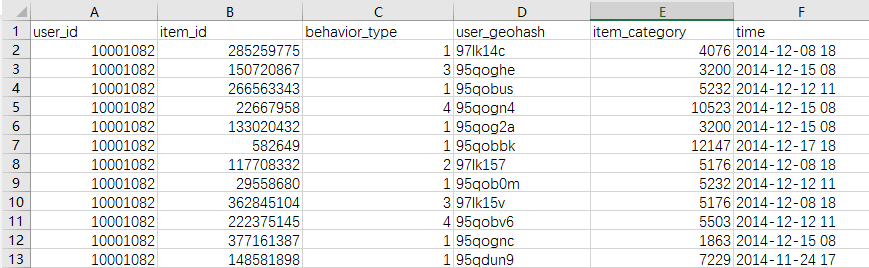# python3怎么筛选excel中特定的行（行中的值满足某个条件/行中的值属于某个集合）

在这之前我们先介绍一个pandas里面一个函数 loc（）

英文解释是这样的：Purely label-location based indexer for selection by label.

.loc[] is primarily label based, but may also be used with a boolean array.

最通俗的讲就是标签索引器问题一：我们想要“behavior_type”列中所有为3或4的行

import pandas as pd
import numpy as np

import csv

print(df.loc[df['behavior_type']>1,:])

print(df.loc[df['behavior_type'].isin([3,4]),:]) 注意 这里的isin（[ ]）有小括号 也有中括号集合的形式。

print(df[df['behavior_type'].isin([3,4])])

print(df.loc[df['user_geohash'].str.contains('qo')])

df是一个dataframe，列名为A B C D
具体值如下：
A    B    C     D
0    ss   小红  8
1    aa   小明  d
4    f          f
6    ak   小紫  7
dataframe里的属性是不定的，空值默认为NA。
一、选取标签为A和C的列，并且选完类型还是dataframe
df = df.loc[:, ['A', 'C']]
df = df.iloc[:, [0, 2]]
二、选取标签为C并且只取前两行，选完类型还是dataframe
df = df.loc[0:2, ['A', 'C']]
df = df.iloc[0:2, [0, 2]]